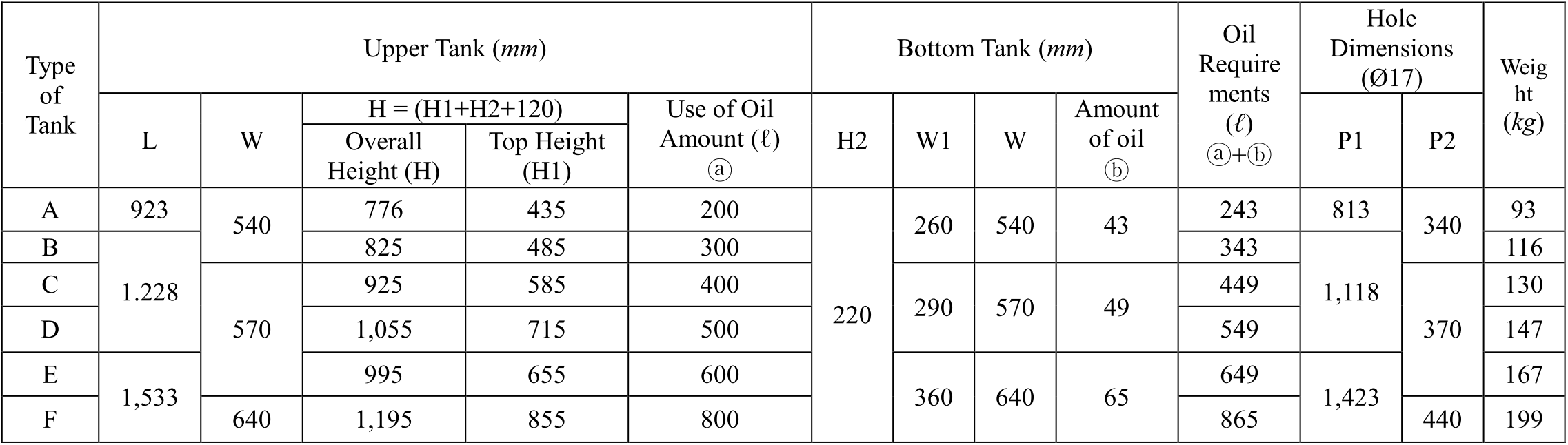## Selection of Hydraulic Unit and Tank Specifications

A Neccessary Amount Calculation and Selection of Hydraulic Oil Tank

➞ The amount of oil is calculated with a diameter and length of cylinder.Hydraulic Tank DimensionsUse of oil amount (l):   = (L1 x W x H1) / 1000

Basic needs (l):             = (L1 x W1 x H2) / 1000

Oil requirements (l)=   ⓐ (use of oil amount) + (basic needs)